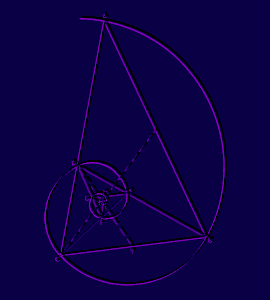FIBONACCI SEQUENCEFIBONACCI SEQUENCE

If we have a sequence of numbers such as 2, 4, 6, 8, ... it is called an arithmetic series . Can you determine the rule to get from one number in the series to the next?

A sequence of numbers such as 2, 4, 8, 16, ... it is called a geometric series . What is the rule to get from one number to the next in this series?Leonardo Fibonacci, who was born in the 12th century, studied a sequence of numbers with a different type of rule for determining the next number in a sequence.
He began the sequence with 0,1, ... and then calculated each successive number from the sum of the previous two.
This sequence of numbers is called the Fibonacci Numbers or Fibonacci Sequence. The Fibonacci numbers are interesting in that they occur throughout both nature and art. Especially of interest is what occurs when we look at the ratios of successive numbers.

1. First, calculate the first 20 numbers in the Fibonacci sequence. Remember that the formula to find the nth term of the sequence (denoted by F[n]) is F[n-1] + F[n-2].

2. Next, look at the ratios found by F[n]/F[n-1]. We'll get you started.
1/1 = 1
2/1 = 2
3/2 = 1.5
5/3 = 1.666...
8/5 = 1.6

Calculate the ratios using all of the Fibonacci numbers you calculated above. What do you notice happens to this ratio as n increases? Does these ratios seem to be converging to any particular number? Graph the ratios and see what they look like. Check your ratios and graph here.

3. Now, consider the ratios found by F[n-1]/F[n], that is the reciprocals of the ratios in exercise 2. above. Graph these results. What do you find? What value do you suspect these ratios are converging to? What does this tell you is a property of the ratios we have found?

Continue on to the next page.